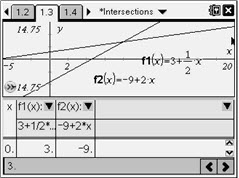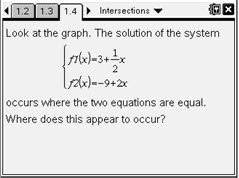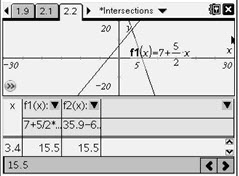# Activities

••• ##### Subject Area

• Math: Algebra I: Linear Functions

• ##### Author9-12

30 Minutes

• ##### Device
• TI-Nspire™
• TI-Nspire™ CAS
• ##### Software

TI-Nspire™
TI-Nspire™ CAS

2.1

## Intersections#### Activity Overview

Students graph two linear functions and estimate the solution of the system graphically using the Graph Trace feature. Then they examine functions tables to find the exact solution of the system.

#### Key Steps

•Students are initially given two functions to graph. They then Trace the graph of f1 and see that the intersection point is approximately (8, 7).

Next they trace f2 and see that the point (8, 7) also lies on its graph. Then they trace both functions at once as see that as the identical x-values of the two points approach 8, their y-values get closer to each other.

•Next students add a function table to their graph and examine it in search of an x-value that gives the same y-value for both functions. This point, (8, 7) is defined as the solution to this system of linear equations.

•Then, students continue to explore with other pairs of functions.